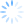Does Excel seem to freeze when using a workbook with a lot of CDXZipStream or CDXStreamer data?  This is often caused by automatic recalculation of worksheet formulas.

Our Microsoft Excel add-ins CDXZipStream and CDXStreamer usually return data in the form of Excel formulas or array formulas.  Array formulas return data in more than one cell, in multiple rows and columns; Excel surrounds the formula with braces ({ }) and places an instance of the formula in each cell of the array range.  Here are the array formula and returned data when using the CDXRadius function, which finds ZIP codes within a radius distance of a target location:When formulas or array formulas include a worksheet cell address, things can get a little complicated.  Excel recalculates all affected formulas whenever a change is made in a worksheet value.  For example, change the target zip code for the CDXRadius function above (in cell A1), and the returned array of ZIP codes within the target radius gets recalculated automatically.

This is generally not a problem when a few values are changed, but making changes to a long address list (by sorting it, for example) can cause a lot of subsequent recalculation in affected formulas or arrays.  The overall process for this can take a very long time, to the point where it seems like the application is frozen and unresponsive. Recalculation also can occur when an old workbook is converted to a new version of Excel.  In later versions of Excel you may see the progress of the recalculations at the bottom of the display.

So what can you do to keep this from happening?  First, if you don’t need formulas in your spreadsheet, get rid of them.  Formulas are great for copying and applying to new data, but if you are done using them you can copy the formula range and paste it back to the same location as values.  The formulas disappear, and no recalculation will occur again.  For more detail on how to do this, please see this short tutorial from Microsoft.

When using the address verification function in CDXStreamer, you can also check the “Set Array to Values” option and all the data will be returned as values, not formulas.

But what if you want the formulas to remain in the worksheet?  In this case you can control when recalculation occurs by turning off automatic recalculation, and then recalculating only when it’s convenient.  In later versions of Excel, click on the File tab (or Office button in Excel 2007), and in the Options area select Formulas, and select the desired setting under Workbook Calculation.   Then just use CTRL-ALT-F9, which will force recalculation of all worksheets in all open workbooks.

Whenever you have of data and related formulas in a worksheet, manual recalculation can save you a lot of waiting time.  Consider turning off automatic recalculation (whether you’re using our add-ins or not) to be in full control of your worksheet data and make your Excel sessions less frustrating and more efficient.  For more information on using manual recalculation in all Excel versions, please see this Microsoft article.